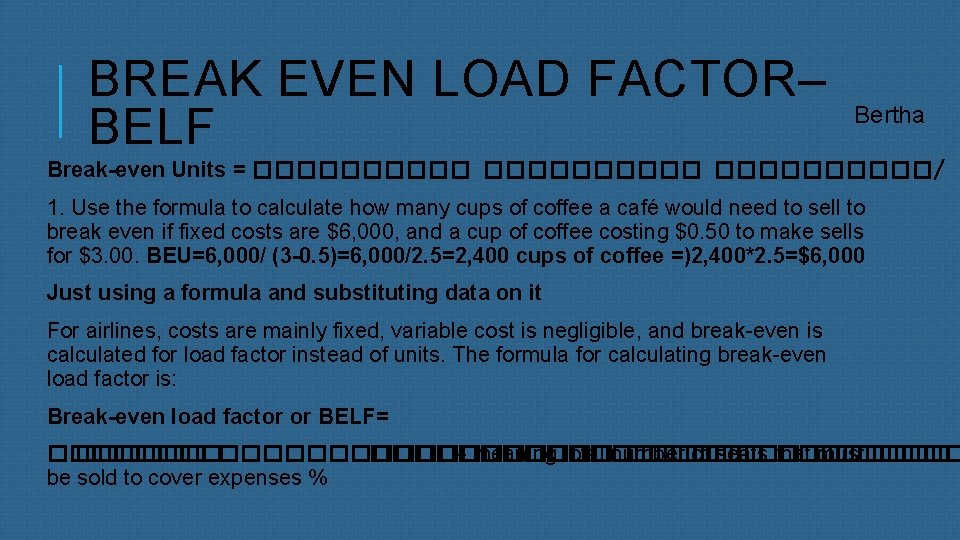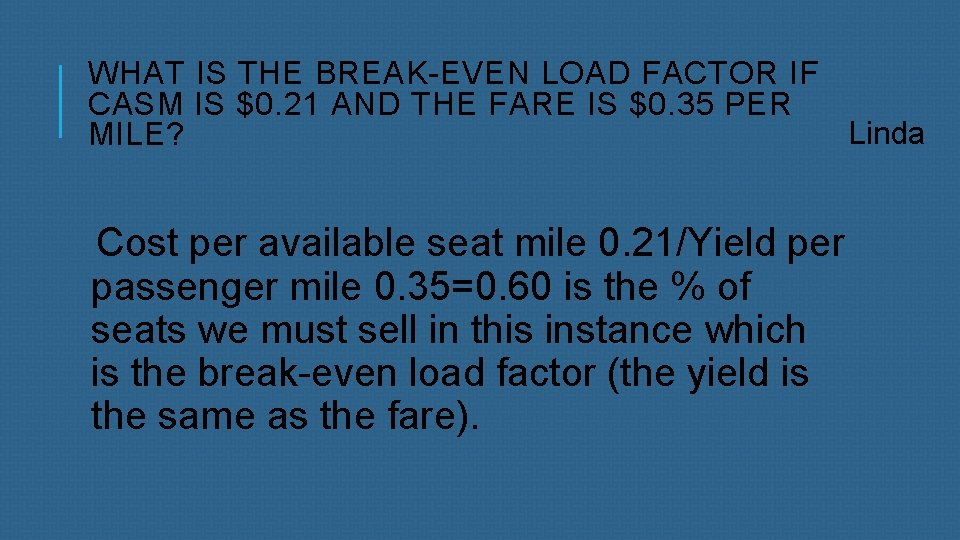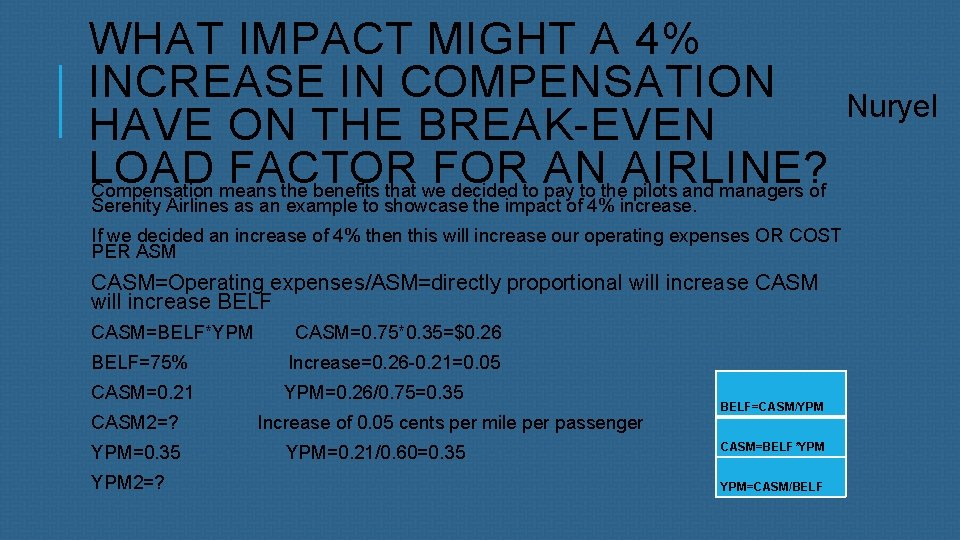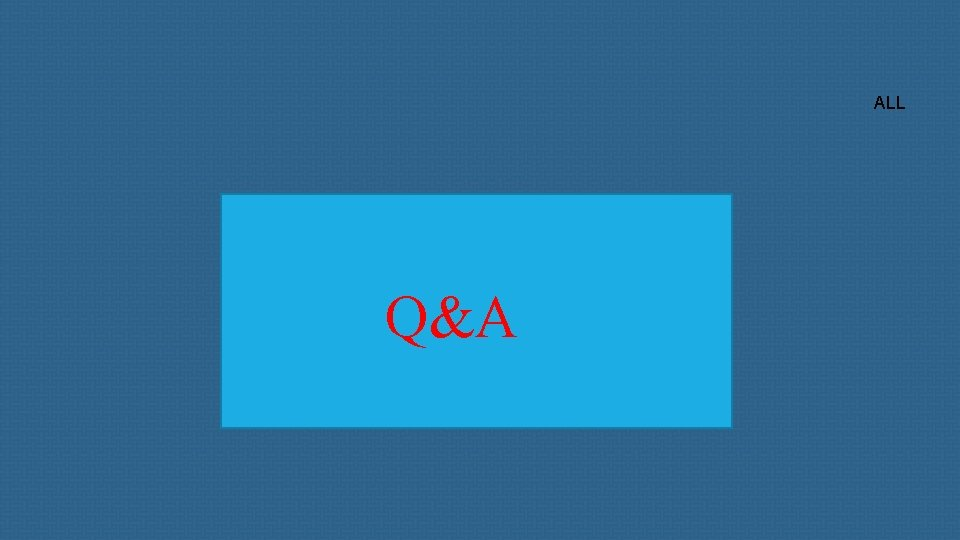# SERENITY AIRLINES Break Even Analysis BREAK EVEN LOAD

• Slides: 9SERENITY AIRLINES Break Even AnalysisBREAK EVEN LOAD FACTOR– BELF Bertha Break-even Units = ����������/ ( 1. Use the formula to calculate how many cups of coffee a café would need to sell to break even if fixed costs are \$6, 000, and a cup of coffee costing \$0. 50 to make sells for \$3. 00. BEU=6, 000/ (3 -0. 5)=6, 000/2. 5=2, 400 cups of coffee =)2, 400*2. 5=\$6, 000 Just using a formula and substituting data on it For airlines, costs are mainly fixed, variable cost is negligible, and break-even is calculated for load factor instead of units. The formula for calculating break-even load factor is: Break-even load factor or BELF= ������������� – meaning total number ���� of seats that ������� must be sold to cover expenses %FORMULA FOR CASM=TOTAL OPERATING COST/ASM Total operating cost=\$1. 6 M ASM or available seat mile=8. 5 M Given total operating cost of \$1. 6 million and 8. 5 million available seat miles, calculate the cost per available seat mile (CASM)=1. 6/8. 5=\$0. 19 ¢ CASM is expressed in cents to operate each seat mile offered, and is determined by dividing operating costs by ASM BerthaWHAT IS THE BREAK-EVEN LOAD FACTOR IF CASM IS \$0. 21 AND THE FARE IS \$0. 35 PER Linda MILE? Cost per available seat mile 0. 21/Yield per passenger mile 0. 35=0. 60 is the % of seats we must sell in this instance which is the break-even load factor (the yield is the same as the fare).IN AIRLINE, FARE SALES WILL REDUCE THE YIELD. YOU CAN EXPECT YIELD PER PASSENGER MILE TO DROP ABOUT 10% FOR Linda EACH MONTH OF FARE SALE. CALCULATE THE BREAK-EVEN LOAD FACTOR IS CASM IS \$0. 21, REGULAR FARE IS \$0. 35 PER MILE, AND YOU ARE OFFERING A TWO-MONTH FARE Yield=. 35*(10*2)=. 07=. 35 -. 07=0. 28 is the new yield with the discount Now, the BELF=0. 21/. 28=0. 75=75% which is conclusive because you need more seats occupied or sold. Indirectly proportional as per formula BELF=CASM/YPMWHAT IMPACT MIGHT A 4% INCREASE IN COMPENSATION HAVE ON THE BREAK-EVEN LOAD FACTOR FOR AN AIRLINE? Compensation means the benefits that we decided to pay to the pilots and managers of Serenity Airlines as an example to showcase the impact of 4% increase. If we decided an increase of 4% then this will increase our operating expenses OR COST PER ASM CASM=Operating expenses/ASM=directly proportional will increase CASM will increase BELF CASM=BELF*YPM CASM=0. 75*0. 35=\$0. 26 BELF=75% Increase=0. 26 -0. 21=0. 05 CASM=0. 21 YPM=0. 26/0. 75=0. 35 CASM 2=? YPM=0. 35 YPM 2=? Increase of 0. 05 cents per mile per passenger YPM=0. 21/0. 60=0. 35 BELF=CASM/YPM CASM=BELF*YPM YPM=CASM/BELF NuryelINCIDENT REPORT: DUAL DESIGNATE Option three will leave the company open to passengers with connecting flights as well as being able to maintain the autonomy and business structure. Julian120, 000 FOR 4 AIRCRAFTS 2 YEARS TO PAY CHART JulianALL Q&A